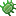###Issue: Actuate Back Easing Bug

Pages: 1

#### sundaefactory

• Subscriber - Stencyl Indie
•October 07, 2015, 08:20:18 am
I think there is a bug with the actuate lib used in Stencyl

The current code in Back.hx, line 86 is
Code: [Select]
`public function calculate (k:Float):Float {   if ((k /= 0.5) < 1) return 0.5 * (k * k * (((s *= (1.525)) + 1) * k - s));   return 0.5 * ((k -= 2) * k * (((s *= (1.525)) + 1) * k + s) + 2);}public function ease (t:Float, b:Float, c:Float, d:Float):Float {   if ((t/=d/2) < 1) return c/2*(t*t*(((s*=(1.525))+1)*t - s)) + b;   return c/2*((t-=2)*t*(((s*=(1.525))+1)*t + s) + 2) + b;}`
The "s*=" is not right because s is also used in the same expression

This code is working:
Code: [Select]
`public function calculate (k:Float):Float {   var m:Float = s * 1.525;   if ((k /= 0.5) < 1) return 0.5 * (k * k * ((m + 1) * k - m));   return 0.5 * ((k -= 2) * k * ((m + 1) * k + m) + 2);}public function ease (t:Float, b:Float, c:Float, d:Float):Float {   var m:Float = s * 1.525;   if ((t/=0.5*d) < 1) return 0.5 * c * (t*t*((m+1)*t - m)) + b;   return 0.5 * c * ((t-=2)*t*((m+1)*t + m) + 2) + b;}`

#### captaincomic

• Master Stencyler
•October 27, 2015, 05:22:51 pm
Thanks for the patch. If it's a bug in actuate it would be best to report it here: https://github.com/openfl/actuate
When the bug is fixed upstream, we can update our version of the lib.

Anyway, could you describe what is not working with the current code?
• Status changed from New to Need Info
• Tags added: openfl

#### sundaefactory

• Subscriber - Stencyl Indie
•October 28, 2015, 12:41:57 am
ok, I will report it on actuate directly

about the bug, in the expression
Code: [Select]
`0.5 * ((k -= 2) * k * (((s *= (1.525)) + 1) * k + s) + 2);`s is multiplied by 1.525 during the evaluation of a first part and instead of evaluating the original s value in the second part, it's the multiplied value

I just correct the bug by introducing a local variable holding the multiplied value

#### captaincomic

• Master Stencyler
•November 03, 2015, 04:37:35 pm
What I meant is, is it not moving as expected? Wrong direction? To fast/slow?

#### sundaefactory

• Subscriber - Stencyl Indie
•November 03, 2015, 10:10:20 pm
I think you have nothing to expect from this code, the result can be far from the expected value. For example I wanted to use for a slight grow on a small actor and the scale become so big, the actor was bigger than the screen.

#### captaincomic

• Master Stencyler
•November 15, 2015, 08:29:05 am
Tbh, I don't see a problem with it. Could you attach a test game?

##### Issue updated by captaincomic - December 14, 2015, 11:18:05 am
• Tags added: abandoned
• Status changed from Need Info to Closed

Pages: 1

### Details

• Reported
October 07, 2015, 08:20:18 am
• Updated
December 14, 2015, 11:18:05 am

• View Status
Public
• Type
Bug
• Status
Closed
• Priority
Normal
• Version
Stencyl 3.3
• Fixed in
(none)
• Assigned to
(none)
• Category
Engine